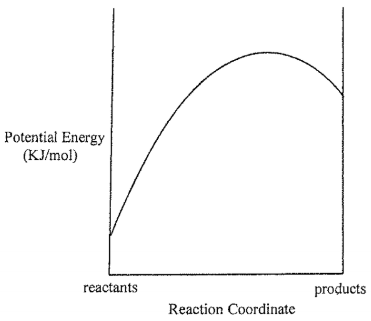# Problem: Consider the Potential Energy vs. Reaction Coordinate diagram shown to the right. For each of the following questions, circle the best answer.If the temperature is raised, i) the rate constant for the forward  reaction will increase more than the rate constant for the back reactionii) the rate constant for the back reaction will increase more than the rate constant for the forward reactioniii) both rate constant will increase to the same extent

###### FREE Expert Solution

For this problem, we have to determine which among the statement is true when the temperature is raised

• In this case, we can estimate the sign of ΔH by using the reaction diagram provided
80% (378 ratings)###### Problem Details

Consider the Potential Energy vs. Reaction Coordinate diagram shown to the right. For each of the following questions, circle the best answer.If the temperature is raised,

i) the rate constant for the forward  reaction will increase more than the rate constant for the back reaction

ii) the rate constant for the back reaction will increase more than the rate constant for the forward reaction

iii) both rate constant will increase to the same extent

Frequently Asked Questions

What scientific concept do you need to know in order to solve this problem?

Our tutors have indicated that to solve this problem you will need to apply the Energy Diagram concept. If you need more Energy Diagram practice, you can also practice Energy Diagram practice problems.

What is the difficulty of this problem?

Our tutors rated the difficulty ofConsider the Potential Energy vs. Reaction Coordinate diagra...as medium difficulty.